# Ex.6.4 Q4 Squares and Square Roots - NCERT Maths Class 8

Go back to  'Ex.6.4'

## Question

Find the least number which must be subtracted from each of the following numbers so as to get a perfect square. Also find the square root of the perfect square so obtained.

(i) $$402$$

(ii) $$1989$$

(iii) $$3250$$

(iv) $$825$$

(v) $$4000$$

## Text Solution

What is known?

Numbers that are not perfect square

What is unknown?

What must be subtracted from the numbers so as to get perfect square

Steps

(i)

Square root of $$402$$ is calculated as: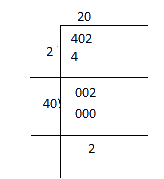It is evident that square of $$20$$ is less than $$402$$ by $$2$$. If we subtract the remainder from the number, we get a perfect square. Therefore, the required perfect square is

Therefore, required perfect square $$= 402 − 2 = 400$$

$$402 - 2 = 400\;{\rm{and}}\;\sqrt {400}= 20$$

(ii)

Square root of $$1989$$ by long division method.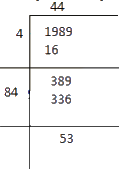The remainder obtained is $$53$$. The square of $$44$$ is less than the given number $$1989$$ by $$53$$. Therefore, perfect square can be obtained by subtracting $$53$$ from the given number $$1989$$.

Therefore, required perfect square $$= 1989 − 53 = 1936$$

$${\rm{And}}\;\sqrt {1936}= 44$$

(iii)

Square root of $$3250$$ can be calculated by long division method as follows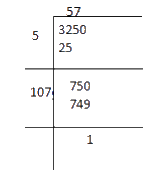The remainder obtained is $$1$$. The square of $$57$$ is less than $$3250$$ by $$1$$. Therefore, the required perfect square  $$= 3250 − 1 = 3249$$

$${\rm{And}}\;\sqrt {3249}= 57$$

(iv)

Square root of $$825$$ can be calculated by the long division method as follows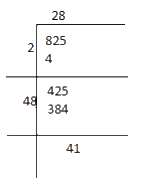The remainder is $$41$$.it shows that the square of $$28$$ is less than $$825$$ by $$41$$.

Therefore, required perfect square $$= 825 − 41 = 784$$

$${\rm{}}\;\sqrt {784}= 28$$

(v)

Square root of $$4000$$ can be calculated by the long division method as follows.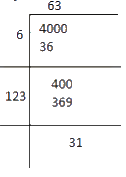The remainder is $$31$$, it represents that the square of $$63$$ is less than $$4000$$ by $$31.$$ Therefore, the square perfect square $$= 4000 − 31 = 3969$$.

$$\sqrt {3969}= 63$$

Learn from the best math teachers and top your exams

• Live one on one classroom and doubt clearing
• Practice worksheets in and after class for conceptual clarity
• Personalized curriculum to keep up with school